# Algebra II : Understanding Radicals

## Example Questions

### Example Question #10 : Radicals As Exponents

Simplify: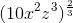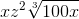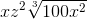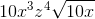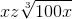Explanation:

To simplify the expression, we must remember that a fraction as a power denotes a radical: the numerator is the power to which the term is taken inside the radical, and the denominator denotes the degree of the root (i.e. 2 means square root, 3 means cube root, etc.)

Rewriting our expression, we get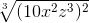which expanded becomes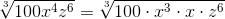Now, move the cubes outside of the cube root, after taking their cube root, leaving behind the terms that aren't cubes:### Example Question #61 : Radicals

Simplify: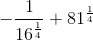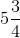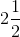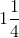Explanation:

The fractional exponent represents the fourth root of the given number.

Evaluate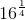.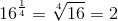Evaluate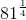.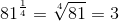Rewrite the fractions.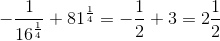The answer is:### Example Question #62 : Radicals

Rewrite the following expression as a radical: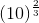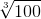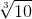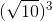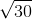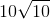Explanation:

The denominator of the exponent represents the root and the numerator represents the power of what the radical is raised to.

We can also separate the powers using the product rule of exponents.

Rewrite the expression as follows: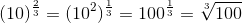Note that the powers in the product can also be interchanged.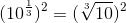The answer is:### Example Question #63 : Radicals

Rewrite the radical as an exponent: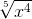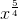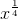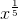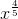Explanation:

In order to rewrite a radical as an exponent, the number in the radical that indicates the root, gets written as a fractional exponent as shown below: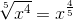In this case, we are done because there are no further simplification steps.

### Example Question #64 : Radicals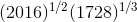None of these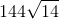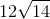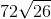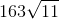Explanation: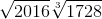Factoring: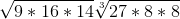Simplifying: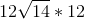### Example Question #65 : Radicals

Rewrite the following radical as an exponent: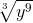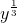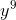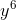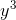Explanation:

In order to rewrite a radical as an exponent, the number in the radical that indicates the root, gets written as a fractional exponent. Distribute the exponent to the term by multiplying it by the exponent of the term as shown below: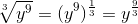From this point simplify the exponent accordingly: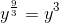### Example Question #66 : Radicals

Rewrite the following radical as an exponent: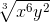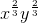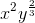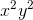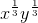Explanation:

In order to rewrite a radical as an exponent, the number in the radical that indicates the root, gets written as a fractional exponent. Distribute the exponent to both terms by multiplying it by the exponents of each term as shown below: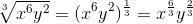From this point simplify the exponents accordingly: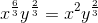### Example Question #67 : Radicals

Rewrite the following radical as an exponent: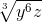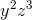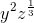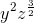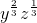Explanation:

In order to rewrite a radical as an exponent, the number in the radical that indicates the root, gets written as a fractional exponent. Distribute the exponent to both terms by multiplying it by the exponents of each term as shown below: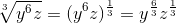From this point simplify the exponents accordingly: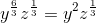### Example Question #68 : Radicals

Rewrite the following radical as an exponent: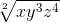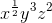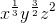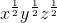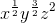Explanation:

In order to rewrite a radical as an exponent, the number in the radical that indicates the root, gets written as a fractional exponent. Distribute the exponent to all terms by multiplying it by the exponents of each term as shown below: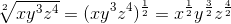From this point simplify the exponents accordingly: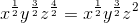### Example Question #69 : Radicals

Rewrite the following radical as an exponent: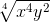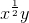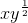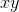Explanation:

In order to rewrite a radical as an exponent, the number in the radical that indicates the root, gets written as a fractional exponent. Distribute the exponent to all terms by multiplying it by the exponents of each term as shown below: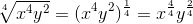From this point simplify the exponents accordingly: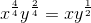### All Algebra II Resources